﻿ 基于锂电池–超级电容器混合储能的配电网广域测量装置电源研究 Research on Power Supply of Wide-Area Measurement Device in Distribution Network Based on Mixed Energy Storage of Lithium Battery and Super Capacitor

Transmission and Distribution Engineering and Technology
Vol. 08  No. 04 ( 2019 ), Article ID: 33368 , 11 pages
10.12677/TDET.2019.84015

Research on Power Supply of Wide-Area Measurement Device in Distribution Network Based on Mixed Energy Storage of Lithium Battery and Super Capacitor

Kai Chen1, Zhonghan Peng1, Qirui Wu1, Jinliang Hu1, Mu Li1, Kaikai Gu1, Ran Gu1, Haikuo Zhou2

1State Grid Electric Power Research Institute Wuhan Nari Limited Liability Company, Wuhan Hubei

2School of Electrical Engineering and Automation, Wuhan University, Wuhan Hubei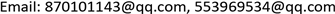Received: Nov. 18th, 2019; accepted: Dec. 3rd, 2019; published: Dec. 10th, 2019ABSTRACT

The development of wide-area synchronous measurement system (WAMS) for distribution network plays an important role in the safe operation of distribution network. In this paper, the functional requirements and power consumption characteristics of distribution network WAMS are analyzed. The low power consumption design is carried out from two aspects: hardware design and software control strategy. In view of the shortcomings of power supply in WAMS device, a new power supply scheme suitable for distribution network WAMS device is proposed. Current transducer (CT) is used as the main power supply, the lithium battery and the supercapacitor are used as the energy element and power element respectively. The test results show that: the composite power supply can supply stable power to the measuring device when the load current of the line is lower than 30 A or power failure.

Keywords:Wide Area Synchronous Measurement, CT Energy Acquisition, Lithium Battery, Supercapacitor, Hybrid Energy Storage

1国网电力科学研究院武汉南瑞有限责任公司，湖北 武汉

2武汉大学电气与自动化学院，湖北 武汉1. 引言

2. 配电网WAMS监测装置结构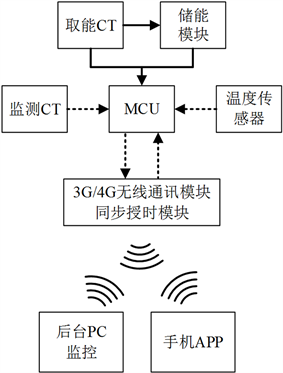Figure 1. The functional structure of distribution network WAMS monitoring device

3. 负载功率需求分析Table 1. Main function module power characteristics of monitoring device

4. 低功耗软件控制策略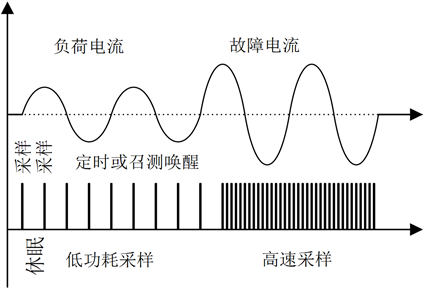Figure 2. Low power sampling strategy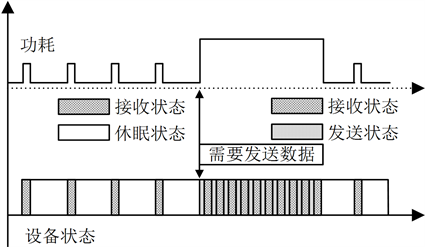Figure 3. Low power data transmission strategy

5. 低功耗软件控制策略

5.1. 储能电池类型选择

Table 2. Characteristics of common energy storage device

5.2. 供电电源设计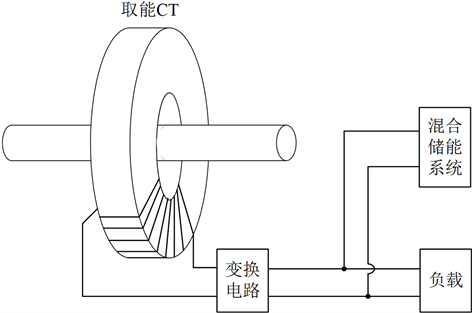Figure 4. Power supply structure of distribution network WAMS monitoring device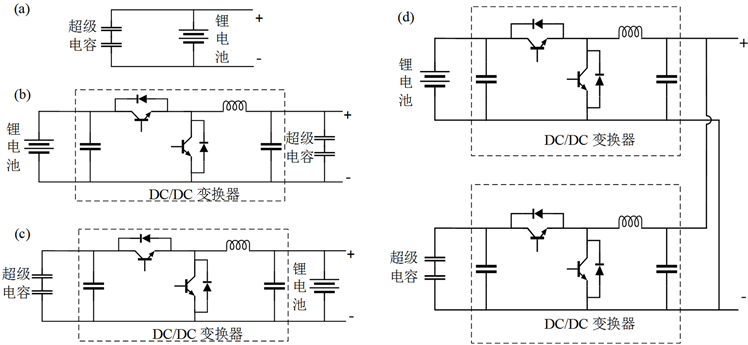Figure 5. Topological of hybrid energy storage of lithium battery and supercapacitor

5.3. 储能容量选择

${C}_{B}=\frac{Pt}{{U}_{S}{S}_{1}{S}_{2}{\eta }_{DC1}}$ (1)

${W}_{k}=\frac{1}{2}\cdot \frac{I{U}_{s}t}{{\eta }_{DC2}}$ (2)

${W}_{s}=\frac{1}{2}\cdot \frac{{Q}^{2}}{C}=\frac{1}{2}C{U}^{2}$ (3)

$\Delta W=\frac{1}{2}C\left({U}_{\mathrm{max}}^{2}-{U}_{\mathrm{min}}^{2}\right)$ (4)

${C}_{SC}=\frac{2\cdot \Delta W}{{U}_{\mathrm{max}}^{2}-{U}_{\mathrm{min}}^{2}}$ (5)

6. 实验验证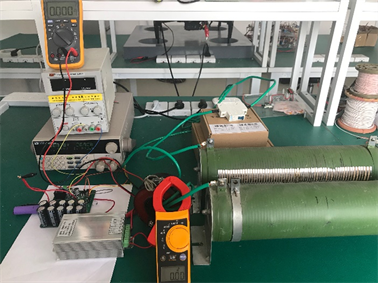Figure 6. Experimental platform of CT energy harvesting and energy storage coupling systemTable 3. Power distribution of distribution network WAMS device

7. 总结

Research on Power Supply of Wide-Area Measurement Device in Distribution Network Based on Mixed Energy Storage of Lithium Battery and Super Capacitor[J]. 输配电工程与技术, 2019, 08(04): 120-130. https://doi.org/10.12677/TDET.2019.84015

1. 1. 吕健双, 李健, 罗超. 不均匀冰对重冰区特高压输电线路对地及交叉跨越距离的影响分析与研究[J]. 陕西电力, 2017, 45(3): 35-38.

2. 2. 吴巧玲, 缪希仁, 叶鎏芳. 高压输电线路短路故障早期检测研究[J]. 电力系统保护与控制, 2016, 44(12): 50-55.

3. 3. 刘宏森, 李迎华, 王恒, 等. 基于实时小波变换的配网短路故障快速检测技术[J]. 高压电器, 2018, 54(3): 225-230, 237.

4. 4. 刘胜波, 阳林, 郝艳捧, 等. 中国沿海地区电网覆冰灾害风险评估[J]. 广东电力, 2017, 30(12): 1-6.

5. 5. 黄新波, 蒋兴良. 智能电网输电线路在线监测技术进展[J]. 广东电力, 2014, 27(6): 72-76.

6. 6. 李震宇, 武国亮, 王志利, 等. 电力微气象风偏灾害监测预警技术及系统实现[J]. 电力系统保护与控制, 2017, 45(1): 125-131.

7. 7. 赵东生, 戴栋, 李立浧, 等. 变压器的阻抗变换特性在交流输电线路杆塔侧电场能采集中的应用[J]. 高电压技术, 2015, 41(12): 3967-3972.

8. 8. 朱永灿, 黄新波, 张冠军, 等. 输电线路在线监测设备供电电源应用分析[J]. 高压电器, 2018, 54(7): 231-236.

9. 9. 郭屾, 王鹏, 张冀川, 等. 高压输电系统电磁能量收集与存储技术综述[J]. 储能科学与技术, 2019, 8(1): 32-46.

10. 10. 胡长春. 10kV电压互感器运行故障原因分析及改进措施[J]. 中国新技术新产品, 2018(24): 91-93.

11. 11. 涂刚. 10kV电压互感器损坏原因分析及防范措施[J]. 电工技术, 2018(19): 96-97, 99.

12. 12. 王玮, 贾明娜, 张新慧, 等. 一种电子式电流互感器的高压侧设计[J]. 自动化仪表, 2016, 37(1): 81-84.

13. 13. 王黎明, 李海东, 陈昌龙, 等. 新型高压输电线路低下限死区大功率在线取能装置[J]. 高电压技术, 2014, 40(2): 344-352.

14. 14. 赵强松, 叶永强, 徐国峰, 等. 一种适用于小电流母线的电子式电流互感器供电电源[J]. 电力系统自动化, 2015(19): 121-125.

15. 15. Li, P., Wen, Y., Zhang, Z., et al. (2015) A High-Efficiency Management Circuit Using Multiwinding Upconversion Current Transformer for Power-Line Energy Harvesting. IEEE Transactions on Industrial Electronics, 62, 6327-6335.
https://doi.org/10.1109/TIE.2015.2431648

16. 16. 李衍川, 江和. 新型高压侧自供电电源设计与研究[J]. 电工电能新技术, 2014(8): 77-80.

17. 17. 赵东生, 戴栋, 邓红雷, 等. 交流输电线路杆塔侧的电势能采集可行性研究[J]. 华南理工大学学报(自然科学版), 2015(4): 119-125.

18. 18. 李岩, 滕云, 冷欧阳, 等. 数据驱动的输电线路在线监测装置可靠性评估[J]. 中国电机工程学报, 2018, 38(15): 4410-4419, 4641.

19. 19. 胡中华, 荣海春, 吴有恒, 等. 超级电容在无人机电气系统中的应用探讨[J]. 自动化与仪表, 2018, 33(9): 99-103.

20. 20. 李泉. 锂离子动力电池管理系统关键技术研究[D]: [博士学位论文]. 长沙: 湖南大学, 2017.

21. 21. 刘云鹏, 李雪, 韩颖慧, 等. 锂离子超级电容器电极材料研究进展[J]. 高电压技术, 2018, 44(4): 1140-1148.

22. 22. 周健瑶. 结合超级电容和锂电池的电流互感器取能电源研究[D]: [硕士学位论文]. 重庆: 重庆大学, 2014.